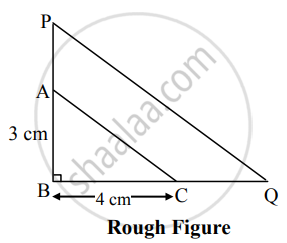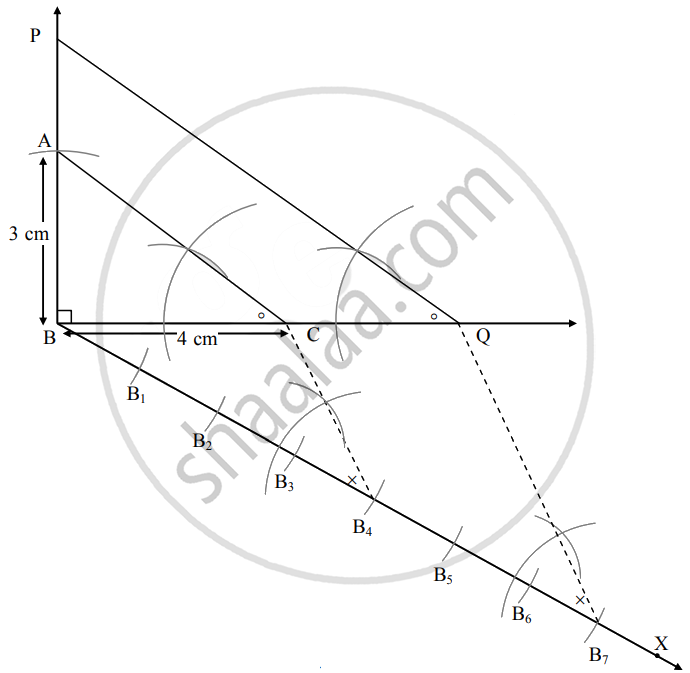# ∆ABC ~ ∆PBQ. In ∆ABC, AB = 3 cm, ∠B = 90°, BC = 4 cm. Ratio of the corresponding sides of two triangles is 7:4. Then construct ∆ABC and ∆PBQ - Geometry

Diagram

∆ABC ~ ∆PBQ. In ∆ABC, AB = 3 cm, ∠B = 90°, BC = 4 cm. Ratio of the corresponding sides of two triangles is 7: 4. Then construct ∆ABC and ∆PBQ

#### SolutionAnalysis: As shown in the figure,

Let B–A–P and B–C–Q.

∆PBQ ∼ ∆ABC

∴ ∠PQB ≅ ∠ACB  .....[Corresponding angles of similar triangles]

"PB"/"AB" = "BQ"/"BC" = "PQ"/"AC"  .....(i) [Corresponding sides of similar triangles]

∴ "PB"/"AB" = "BQ"/"BC" = "PQ"/"AC" = 7/4  ......[Given]

∴ Sides of ∆PBQ are longer than corresponding sides of ∆ABC.

∴ If seg BC is divided into 4 equal parts, then seg BQ will be 7 times each part of seg BC.

So, if we construct ∆ABC point Q will be on side BC, at a distance equal to 7 parts from B.

Now, point P is the point of intersection of ray AB and a line through Q, parallel to AC.

∴ ∆PBQ is the required triangle similar to ∆ABC.Steps of construction:

1. Draw seg BC of length 4 cm.
2. Take ∠B as 90° and draw an arc of 3 cm on it. Name the point as A.
3. Join seg AC to obtain ∆ABC.
4. Draw ray BX such that ∠CBX is an acute angle.
5. Locate points B1, B2, B3, B4, B5, B6, B7 on ray BX such that, BB1 = B1B2 = B2B3 = B3B4 = B4B5 = B5B6 = B6B7.
6. Join point C and B4.
7. Through point, B7 draw a line parallel to seg CB4 which intersects seg BC at point Q.
8. Draw a line parallel to AC through Q to intersect line AB at point P.
∆PBQ is the required triangle similar to ∆ABC.
Concept: Division of a Line Segment
Is there an error in this question or solution?